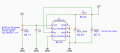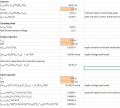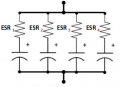# Calculate Total Capacitance and ESR of Parallel Non-ideal Capacitors

#### mriksman

Joined Aug 31, 2010
113
Hi,

Given 2 capacitors; a 330uF with 89mohm ESR, and a 4.2uF with a 7mohm ESR, I'd like to calculate the total capacitance/ESR when in parallel with a 200kHz frequency.

Convert C into X using (1/2*PI*f*C)
X = 0.003215251
Therefore, Impedance Z1 is the ESR for the Real part of Z plus X for the imaginary part.
Z1 = 0.089 - 0.003215j

I perform the same on the other capacitor and get
Z2 = 0.007 - 0.25262j

Then I use the impedance formula for parallel impedance; 1/((1/Z1)+(1/Z2)), and get
Z = 0.07686-0.0295833i
So ESR is the Real(Z) part = 76.86mohm?
And X is the Imaginary(Z) = 0.0295833mohm.
So C is (1/2*PI*f*X) = 35.865uF

That doesn't seem right. There is a 330uF and a 4.7uF in parallel. It doesn't seem right that the total capacitance has dropped to 35.865uF when in parallel.

#### MisterBill2

Joined Jan 23, 2018
16,616
Draw the equivalent circuit with the capacitance and the ESR showing, and then go through the math again. What I see is a logic error, although I have not traced it out yet. The process gives an answer that fails the Maselowski Criteria of "is this answer reasonable."
The fact is that you can not simply add reactance of separate circuits.
One method will be to calculate the current and phase through each capacitor , then sum the currents, and then solve for the equivalent single capacitance to draw that current.
And by the way, this seems a lot like some homework or an exam question.

#### mriksman

Joined Aug 31, 2010
113
Hah. Homework. Not likely.
I am trying to use a Linear Regulator for the first time. And, instead of just doing what the datasheet says, I want to understand the 'why', and how to choose components based on design requirements.MLCCs are great for low ESR, but then I have issues with dealing with voltage droop/transients because of their small sizes. Electrolytics tend to have large ESR, but lots of capacitance. Tantalums sit somewhere in the middle.

I want to do the maths to see what the effective ESR and Capacitance is if I have say, 1 Electrolytic, and 1 or 2 MLCCs in parallel. How do I determine what ripple current the Electrolytic will face (and if it will be spec'd OK). How will I determine the total capacitance so I know the transients equations are met. Using 'ideal' capacitors in parallel, you simply sum the capacitance values. With resistors, is 1/((1/R1)+(1/R2)); but how is the calculation done with the followingSo no. Not homework.

#### MisterBill2

Joined Jan 23, 2018
16,616
OK, and I did not intend that thought to be offensive. But there do appear questions that are definitely homework or tests, or most recently a Master's Thesis .
There is extensive application data available from several of the semiconductor companies and some of it covers the why as well as the what. And since the application data is to help use their products it is quite accurate, totally different from yootoob noise.